Buckwestern.com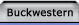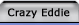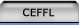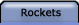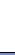Rocket Links Fleet  Pictures  L3 Project  Information  Misc. Links  Motor Codes  TRA/NAR Motor List  NAR  Tripoli Other Links NAR  Tripoli  CBS Sportsline  CNN News  FOX Sports  FOX News  ESPN  Husker Football  Landover Baptist  Stick Death  Suck.com  Best Christmas EVER!  The Onion Weather Links NOAA Weather  CNN Weather  Weather Channel Calendar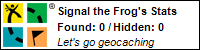Up and Away!Most of the information on these pages is here for my benefit, i.e. there was something I wanted/needed to know, so I gathered that information. I have posted it on the chance that it might be useful to someone else.

Motor Stuff
You can calculate the thrust to weight ratio of a motor using the equation:
Thrust to weight ratio = Motor's average thrust / 4.45 / ratio

 Motor Thrust:

To get the accepted 5:1 thrust to weight ratio of a J330 you divide 330 by 4.45, (the number of Newtons per pound). Divide the result by 5 to get 14.83 Lbs., which is the 5:1 thrust to weight ratio.

If you have built a rocket before choosing an ideal motor, you can also find out what motors will be OK to fly in your rocket by using this equation:

Motor's average thrust = Rocket weight in Lbs * 4.45 * ratio

 Rocket Weight:

So if your rocket weighs in at 15 Lbs. then multiply it by 4.45 and then again by the ratio you want and you'll get a number you can compare to the average thrust of a motor. Any motor with an average thrust greater than the value calculated should be fine.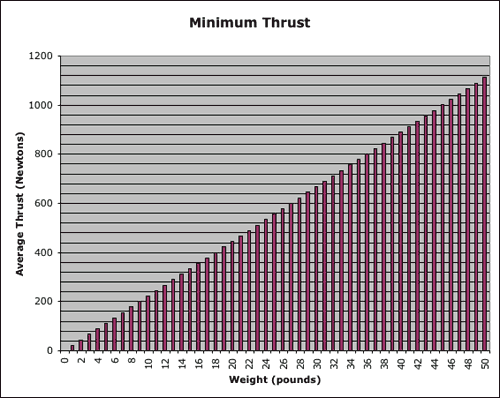Descent Rates and Parachute Sizing

I use a fairly well-published descent calculation:

D = sqrt( (8 m g) / (p r Cd v2) )

Where:

• D is the chute diameter in meters
• m is the rocket mass in kilograms
• g is the acceleration of gravity = 9.8 m/s2
• p is 3.14159265359
• r is the density of air = 1.22 kg/m3
• Cd for the chute, which is 0.75 for a flat sheet parachute, or 1.5 for a hemispherical parachute.
• v = ground impact speed
• Rocket should hit the ground at ~15 fps. 16.4 fps equals a drop from 4'. Drogue chutes descent rates should be around 50 fps.

1 pound = 0.45359237 kilogram

 Rocket Weight (kg):

#### Example

We'll size a parachute for my RCDP Flash-M, which weighs in at 17.5 pounds with 75/6400 case, or 7.955 kg.

• m = 7.955 kg
• Cd = 1.5
• v = 5 m/s

1 inch = 0.0254 meter

D = sqrt((8 m g) / (p r Cd v2)) = sqrt((8*7.955*9.81) / (3.14*1.22*1.5*52)) = 2.08 m

...which equals a 81" parachute.

#### Finding Descent Velocity

We can find the descent velocity, given the chute diameter, by rearranging the equation to get:

v = sqrt((8 m g) / (p r Cd2))

 Rocket Weight (kg): Chute Size (m):

Just some calculations I did out of curiosity since BP Charges were a popular topic of discussion lately.

1 milliliter (ml) = 1 Cubic centimeter (cm3) = 1 gram (water) or 1.0441 grams (Black Powder)

Dr Rocket Motor Fwd Closure BP Charge Wells (if completely full)

29 mm is 1.43 cm3 (ml) (grams more or less)

38 mm is 1.61 cm3 (ml) (grams more or less)

54 mm is 2.94 cm3 (ml) (grams more or less)

29 mm and 38 mm motors have a funnel shaped taper into the hole. Volume and therefore grams is based on the average depth between the shallow part at the edge and the deep part at the middle.

54 mm motors have a flat well.

CTI apparently are all 1.3 g

courtesy of Stan HemphillThis site was created by the Crazy One himself. Crazy Eddie Enterprises, a division of Buckwestern.com